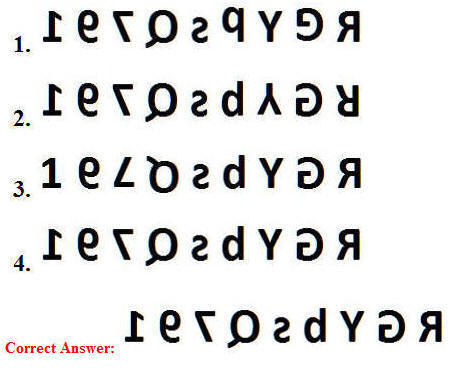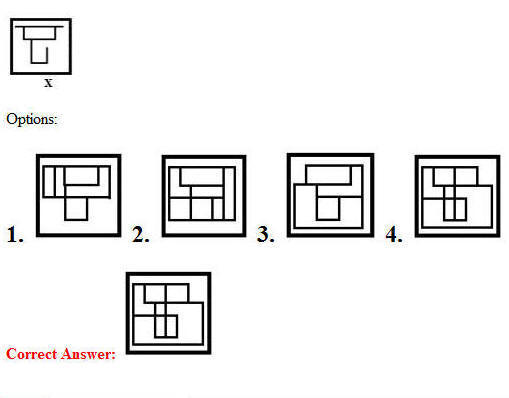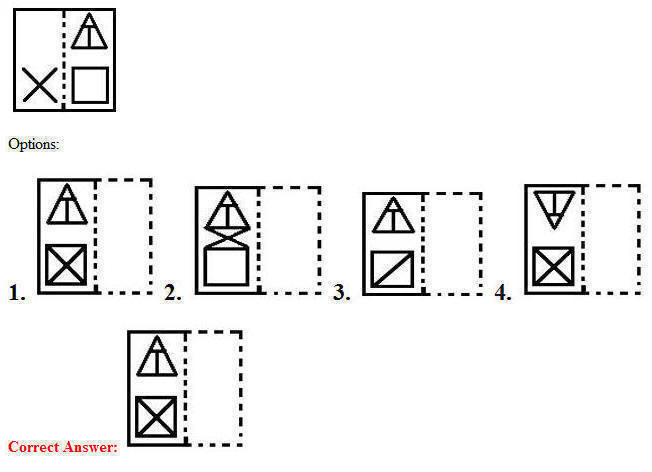# 03 July 2019 – Afternoon Shift (Reasoning)

SSC CHSL (Tier – 1) Online Exam Paper – 2018 “held on 03 JULY 2019”

## Afternoon Shift (Reasoning)

Q1. Select the set in which the numbers are related in the same way as are the numbers of the following set.
(4, 6, 52)

Options:

1. (7, 9, 120)
2. (6, 7, 95)
3. (5, 7, 72)
4. (3, 5, 34)

Q2. Select the correct mirror image of the given combination when the mirror is placed to the right?
R G Y b s q 7 9 1

Options:Q3. Mayank is the son of Chhaya. Chhaya and Deepa are sisters. Gayatri is the mother of Deepa. If Naman is the son of Gayatri, how is Mayank related to Naman?

Options:

1. Nephew
2. Brother
3. Son
4. Father

Q4. Which number will replace the question mark (?) in the following series?
16, 30, 62, 122, 246, ?

Options:

1. 492
2. 488
3. 494
4. 490

Q5. ‘Chassis’ is related to ‘Car’ in the same way as ‘Skeleton’ is related to ‘_________’.

Options:

1. Bones
2. Body
3. Organs
4. Spinal cord

Q6. Select the option in which the given figure is embeddedQ7. Select the option that depicts how the given transparent sheet of paper would appear if is folded at the dotted line.Q8. Select the combination of letters that when sequentially placed in the gaps of the given letter series will complete the series.
_ _ Q D P C _ _ Q C Q _ _ C P D Q C _ D P _ P D

Options:

1. QCPDDPQC
2. QCPCPPQC
3. PCPDDPDC
4. QCDDDPQD

Q9. Three of the following four words are alike in a certain way and one is different. Pick the odd word out.

Options:

1. Organs
2. Atoms
3. Tissues
4. Cells

Q10. Select the option that is related to the third letter-cluster in the same way as the second letter-cluster is related to the first letter-cluster.
QDUJ : VIZO :: LPAR : ?

Options:

1. QUEX
2. QUFW
3. RUFW
4. PUFY

Q11. Select the figure that will come next in the following figure seriesQ12. In the month of June, the number of girls in a class was half of the number of boys. In July, five more girls took admission, whereas five boys left the school. Now, the number of boys and girls is equal in the class. How many students in total were there in the class in June?

Options:

1. 35
2. 30
3. 20
4. 25

Q13. ‘A + B’ means ‘A is the mother of B’.
‘A – B’ means ‘A is the wife of B’.
‘A × B’ means ‘A is the brother of B’.
‘A ÷ B’ means ‘A is the husband of B’.
If T ÷ V + Q – J × M + U, then how is T related to J?

Options:

1. Son-in-law
2. Father-in-law
3. Father
4. Brother

Q14. Select the term that will come next in the following series.
6, 24, 60, 120, ?

Options:

1. 186
2. 210
3. 240
4. 216

Q15. In a code language, CONVEYER is written as FLQYBBBU. How will PERSONAL be written as in that language?

Options:

1. SBUVLQDO
2. RAUVMQYO
3. SBOULQXO
4. SBUVLQXO

Q16. How many triangles are present in the following figure?Options:

1. 25
2. 26
3. 27
4. 28

Q17. Two statements are given, followed by three conclusions numbered I, II and III. Assuming the statements to be true, even if they seem to be at variance with commonly known facts, decide which of the conclusions logically follow(s) from the statements.

Statements:
All parks are schools.
No school is a college.

Conclusions:
I. No college is a school.
II. Some schools are parks.
III. No park is a college.

Options:

1. Only conclusions II and III follow.
2. Only conclusions I and III follow.
3. All the conclusions follow.
4. Only conclusions I and II follow.

Correct Answer: All the conclusions follow.

Q18. Select the Venn Diagram that best illustrate the relationship between the following classes.
Men, Player, Father

Options:Q19. Select the word-pair in which the two words are related in the same way as are the two words in the following word-pair.
Nephrology : Kidney

Options:

1. Cardiology : Liver
2. Ophthalmology : Eyes
3. Zoology : Plants
4. Pathology : Tissues

Q20. Select the set in which the numbers are related in the same way as are the numbers of the following set.
(84, 60, 51)

Options:

1. (80, 98, 69)
2. (64, 80, 72)
3. (52, 64, 47)
4. (72, 56, 62)

Q21. Which two signs should be interchanged in the following equation to make it correct?
868 + 14 × 55 – 1064 ÷ 56 = 117

Options:

1. + and ÷
2. ÷ and ×
3. + and –
4. × and –

Q22. Three Different positions of the same dice are shown. Find the number on the face opposite the face showing ‘3’?Options:

1. 4
2. 5
3. 6
4. 2

Q23. If W = 46 and CAT = 48, then how will you code SALE?

Options:

1. 54
2. 74
3. 19
4. 110

Q24. Select the number-pair in which the two numbers are related in the same way as are the two numbers of the following number-pair.
442 : 21

Options:

1. 238 : 14
2. 223 : 13
3. 257 : 16
4. 323 : 19